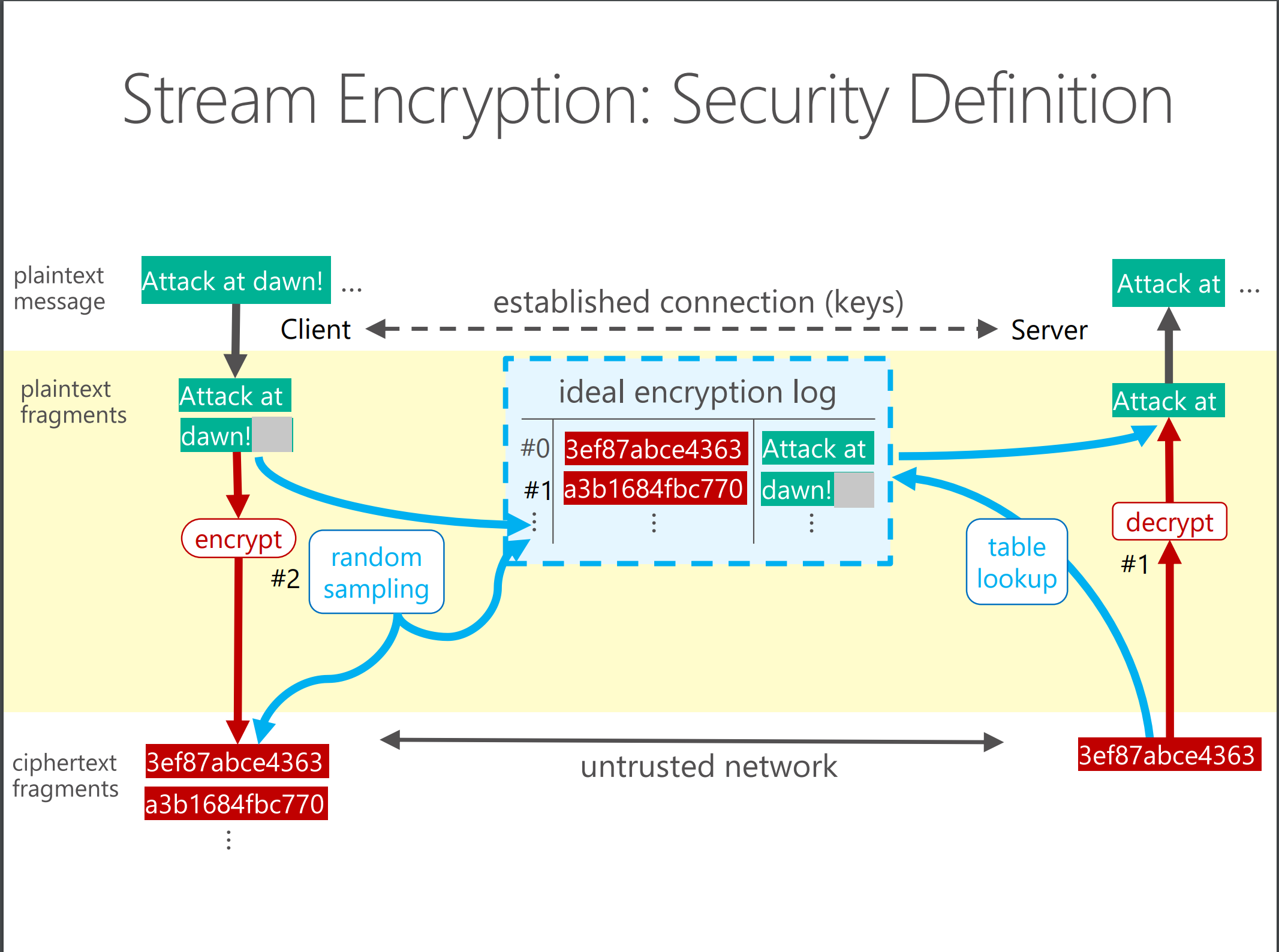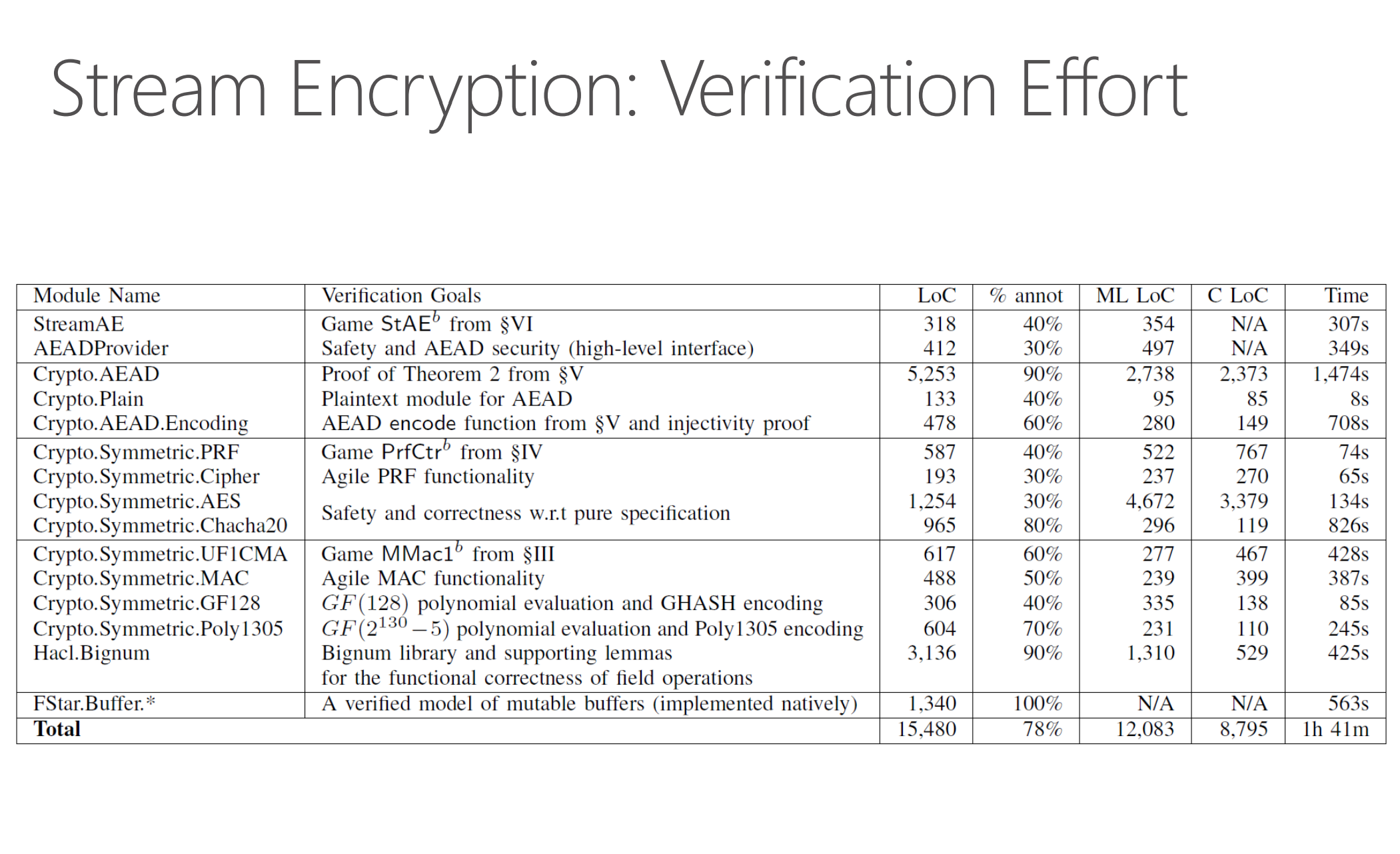## Modeling and Proving Cryptographic Security in F*

### The Setting

• S, R, and A are all programs sharing a communication channel

• A is typically a polynomially bounded computational entity

• Can intercept and tamper with messages on the wire
  Sender (S)   ------network-------->  Receiver (R)
^
|
|
v
Adversary (A)
• Want to make security claims that cover authenticity and secrecy

### Authenticity

When R accepts a message m from S only when S actually sent m

• Correpondence assertions on traces of events
• Event: S:send m or R:receive m
• Trace: Sequence of events
• Authenticity: every R:receive m event in a trace is preceded by a S:send m

### Secrecy

No information about any secrets sent by S is leaked to A

• Secrecy: Usually an equivalence relation on traces
• Messages observed by A are independent of the secrets
• In terms of language-based information-flow securuty
val sender (secret:bytes) (public:bytes) : bytes

let no_leakage = forall s0 s1 p. sender s0 p == sender s1 p

### Perfect Secrecy and Authenticity: Unattainable

• S cannot communicate anything useful about its secret to the R if the messages carries no information about R!

• But, using cryptography, we can prove probabilistic security guarantees

• A cannot distinguish the bytes exhanged by S and R from a random string of bytes, except perhaps with a small probability.

### Cryptographic Games and Hypotheses

• A game G is a program with several procedures and shared state

• Adversary A interacts with G

• A wins if they get G some procedure to emit a specific result (e.g., true)

• Goal: Show that the probability of A winning is low

• I found the following resources useful:

### IND-CPA: INDistinguishability under Chosen-Plaintext Attacks

• Secrecy property for encryption schemes

• Ciphertext leaks only a negligible amount of the plain text
let ind_cpa (encrypt : key -> msg -> cipher) =

(* A secret key and secret bit *)
let key = sample_random_key () in
let b : bool = random_sample_bit () in

(* Encrypt either message, depending on the bit *)
let left_or_right (m0, m1) =
if b
then encrypt key m0
else encrypt key m1 in

(* Check the attacker's guess *)
let check guess = b=guess in

left_or_right,
check
• Attacker's advantage is the probability of guessing right more than half the time

### IND-CPA as an Ideal Functionality

• The definition of IND-CPA is meant to be self-evidently useful notion of ciphertext indistinguishability

• Sometimes, it is possible to prove semantic security (like IND-CPA) of some encryption schemes based on other assumptions

• But, this is out of scope for us

• Usually, one assumes that certain schemes (e.g., AES with IVs) are -IND-CPA, for some symbolic

### UF-CMA: UnForgeability under Chosen-Message Attacks

An authenticity property for Message-Authentication Codes (MACs)

• A MAC: t = mac m k could only be effectively produced through knowledge of the secret key k, i.e., t cannot be forged.

• Authentication by “log lookup”: -UF-CMA secure if the probability of an adversary forging a tag is .

let uf_cma (mac: key -> msg -> tag, verify: key -> msg -> tag -> bool) =x
(* Sample a random key *)
let k = sample_random_key () in
(* Allocate a log *)
let log : ref (list (msg * tag)) = alloc [] in
(* mac wrapper: call mac and log it *)
let mac (m:msg) =
let t = mac m k in
log := (m, t) :: !log;
t in
(* verify wrapper: correct verify using the log *)
let verify (m:msg) (t:tag) =
let b = verify k m t in
b && (m, t) not-in !log in
mac, verify

### Our goal: Authenticated Encryption

• Given an -IND-CPA encryption scheme and a -UF-CMA MAC

We want

• ae_enc that:

• Encrypts the plain text
• MACs the cipher text
• Concatenates the result
• ae_dec that:

• Checks the MAC of the cipher
• Decrypts only authenticated ciphers
• And prove that it is semantically secure with an advantage proportionate to

### HMAC-SHA1

• HMAC-SHA1 as a total function in F*

let hmac_sha1 (k:sha1_key) (m:msg) : tag =
let x5c = byte_of_int 92 in
let x36 = byte_of_int 54 in
let opad = Seq.create blocksize x5c in
let ipad = Seq.create blocksize x36 in
sha1 (xor_key_opad Seq.append
(sha1 (xor_key_ipad Seq.append m)))
• We're going to assume that HMAC-SHA1 is -UF-CMA secure

• But, how best to state that assumption?

### Idealization flags

• Write the concrete and ideal versions of a crypto functionality together
let mac_verify () =
let key = sample_random_key ()
let log = ref [] in

let mac k m =
let t = hmac_sha1 k m in
if Flag.idealizing uf_cma (* BRANCHING HERE IS A CRYPTO HYPOTHESIS *)
then log := (m, t) :: !log;
t
in

let verify k m t =
let ok = (t = hmac_sha1 k m) in
if Flag.idealizing uf_cma (* BRANCHING HERE IS A CRYPTO HYPOTHESIS *)
then ok && (!log contains (m, t))
else ok
in

mac, verify
• mac_verify[uf_cma=true] mac_verify[uf_cma=false]

### Sequences of Games: Multiple Idealizations

concrete_ae
=
ae [ideal_ae=false]
=
mac_verify[uf_cma=false] . enc_dec[ind_cpa=false]
~_e
mac_verify[uf_cma=true] . enc_dec[ind_cpa=false]
~_e'
mac_verify[uf_cma=true] . enc_dec[ind_cpa=true]
=
ae [ideal_ae=true]

### Aside: Secrecy as Parametricity

• val f : 'a -> 'a. What is (f 0)?

• val g : 'a -> int. What is (g 0)? What is (g 1)?

• Well-typed code gains no information about its parametrically typed arguments

### Secrecy as Parametricity, with modules

module Plain
val plain : Type
module Client
val f : plain -> int
• What is Client.f p?
• Not sure, but it's the same as Client.f q

### Modeling confidential plaintexts

• An abstract type of plaintexts Plain.plain
module Plain

val plain : Type

val repr (p:plain{not ind_cpa}) : bytes (* cannot break confidentiality if ind_cpa *)

val coerce (b:bytes{not uf_cma}) : plain (* cannot break integrity if uf_cma *)
• With a function repr that allows breaking confidentiality
• But only when not idealizing for secrecy (i.e. ideal_ind_cpa = false)
• With a function coerce that allows breaking integrity
• But only when not idealizing for authenticity (i.e. ideal_uf_cma = false)

### IND-CPA in F*

• Encryption of zeroes; decryption by table lookup
let ind_cpa () =
let key = sample_random_key () in
let log = ref [] in

let encrypt (p:Plain.plain) : bytes =
let q =
if Idealizing.ind_cpa (*!!! AUDIT, Crypto Hypothesis *)
then zeroes
else Plain.repr p
in
let cipher = encrypt key q in
if ideal_ind_cpa (*!!! AUDIT, Crypto Hypothesis *)
then log := (p, cipher)::!log;
cipher
in

(* MEANT TO BE USED ONLY WITH AUTHENTIC CIPHERS *)
let decrypt (c:cipher) : option Plain.plain =
let q = decrypt key c in
if Idealizing.ind_cpa  (*!!! AUDIT, Crypto Hypothesis *)
then match find !log (fun (_, c') -> c=c') with
| None -> None
| Some (p, _) -> Some p
...

### Streaming Authenticated Encryption### StreamAE Verification